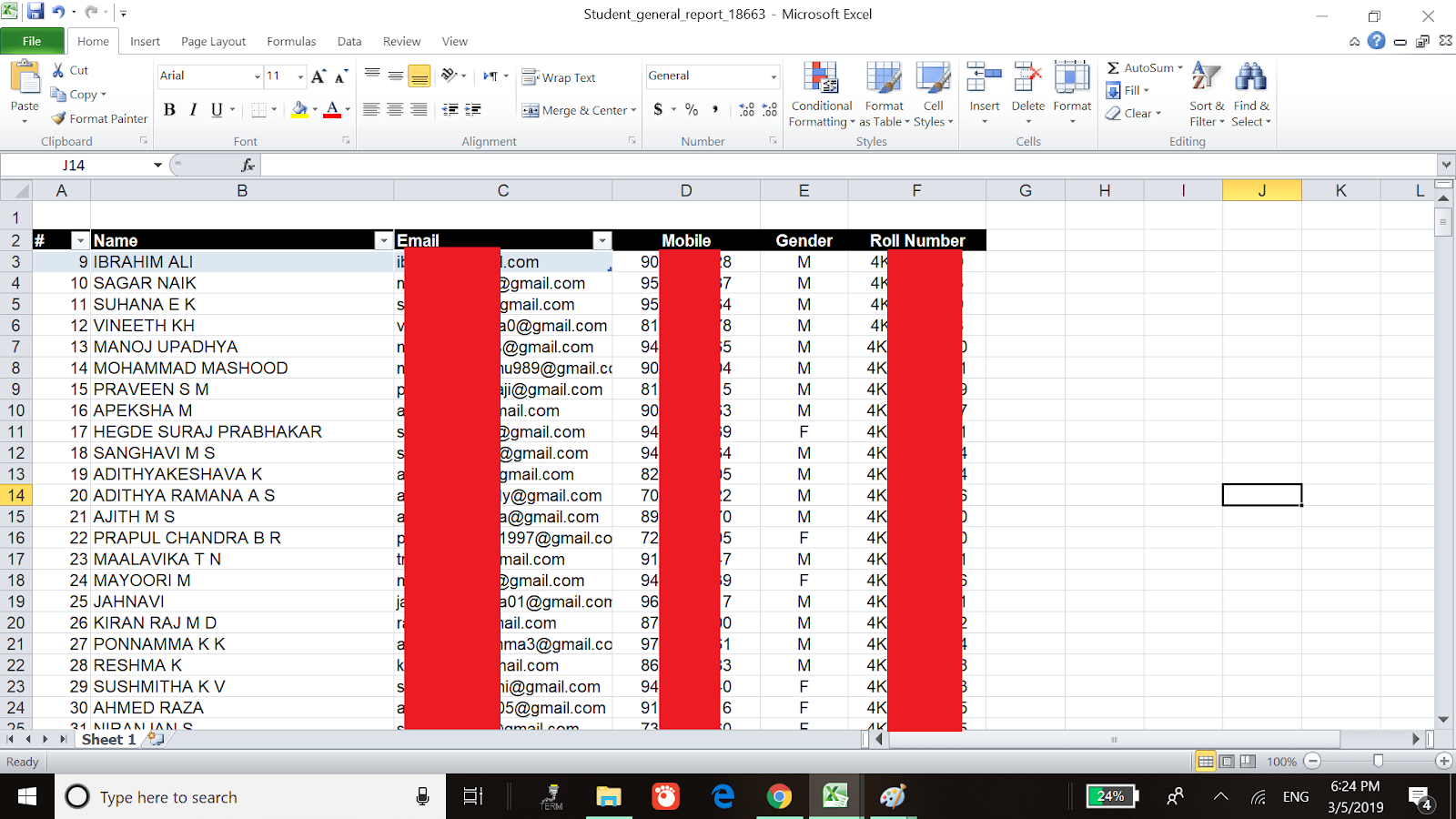Learnyst Support

# How to merge learner data with course/mock test report using VLOOKUP?

In Learner report, you can get details about Learners like their email, phone number, address or any other customer files like roll number or institute name. In course/mock test report, you can find details about course completion data and mock test scores.

The VLOOKUP function in Excel is a tool for looking up a piece of information in a table or data set and extracting some corresponding data/information. In simple terms, the VLOOKUP function says the following to Excel: “Look for this piece of information (e.g., Learner’s email), in this data set (a table), and tell me some corresponding information about it (e.g., Roll number)”.

VLOOKUP formula is given by: VLOOKUP(lookup_value, table_array, col_index_num, [range_lookup])

• Lookup_value– Lookup_value specifies the value that you want to look up for in the first column of a table.

• Table_array– The table array is the data array that is to be searched. The VLOOKUP function searches in the left-most column of this array.

• Col_index_num– This is an integer, specifying the column number of the supplied table_array, that you want to return a value from.

• Range_lookup– This defines what this function should return in the event that it does not find an exact match to the lookup_value. It can be either TRUE or False:

Lookup_value– Lookup_value specifies the value that you want to look up for in the first column of a table.

Table_array– The table array is the data array that is to be searched. The VLOOKUP function searches in the left-most column of this array.

Col_index_num– This is an integer, specifying the column number of the supplied table_array, that you want to return a value from.

Range_lookup– This defines what this function should return in the event that it does not find an exact match to the lookup_value. It can be either TRUE or False:

TRUE– Approximate match, that is, if an exact match is not found, use the closest match below the lookup_value.
FALSE– Exact match, that is, if an exact match not found, then it will return an error.

Steps to use VLOOKUP function to combine Learner’s report data and test report data:

• The image below shows the Learner report data with Gender and Roll Number:

The image below shows the Learner report data with Gender and Roll Number:• Now, to combine Learner report data with Test report data you will have to copy and paste the desired columns with a similar column (Learner’s email) from Learner report to Test report as shown below. With reference to the below example, the orange table is the test report data and the blue table is Learner report data.

Now, to combine Learner report data with Test report data you will have to copy and paste the desired columns with a similar column (Learner’s email) from Learner report to Test report as shown below. With reference to the below example, the orange table is the test report data and the blue table is Learner report data.• Finally, you have to insert the VLOOKUP formula for “Gender”:VLOOKUP([@Email],Table26[#All],2,0) “Rollnumber”:VLOOKUP([@Email],Table26[#All],3,0).

Finally, you have to insert the VLOOKUP formula for “Gender”:VLOOKUP([@Email],Table26[#All],2,0) “Rollnumber”:VLOOKUP([@Email],Table26[#All],3,0).

After adding the VLOOKUP formula the merged data of Learner and Test Report is as shown below:Did this answer your question? Thanks for the feedback There was a problem submitting your feedback. Please try again later.Yes No No results found